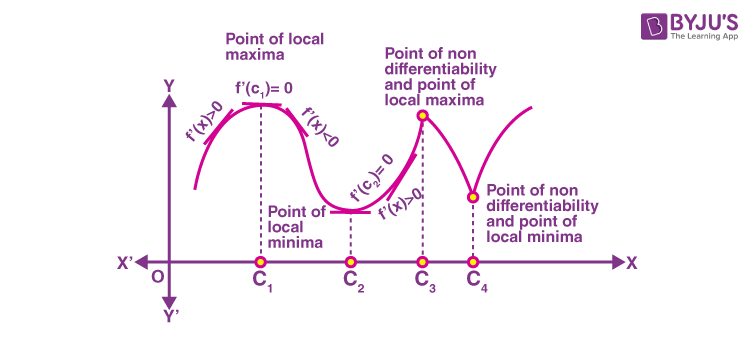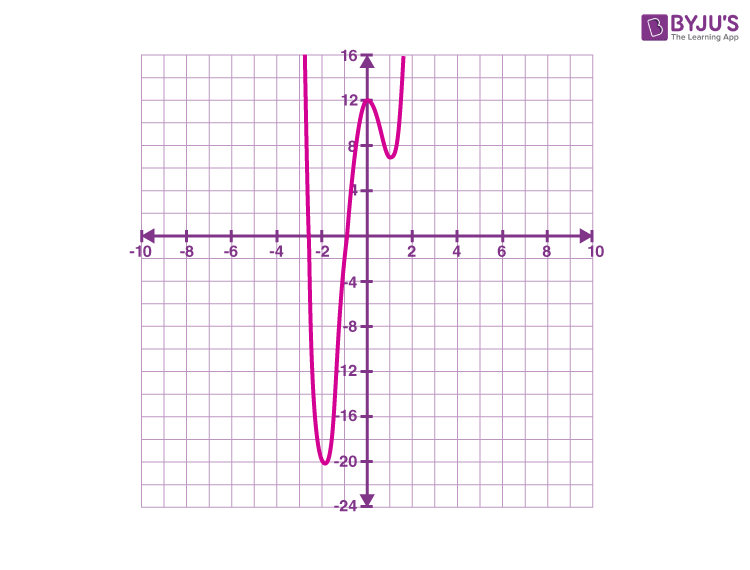# First Derivative Test

We know that every monotonic function assumes its minimum or maximum value at the endpoints of the domain’s definition of the function. A more comprehensive result is “Every continuous function on a closed interval has a minimum value and a maximum value”. A critical point of the function f is the point c in the domain of a function f at which f′(c) = 0 or f is not differentiable. Also, if f is continuous at c and f′(c) = 0, then there exists an h > 0 such that f is differentiable in the interval (c – h, c + h). In this article, you will learn the working rule for finding the points of local maxima or local minima points, i.e. maxima and minima, with the help of only the first-order derivatives.

A derivative test applies the derivatives of a function to determine the critical points and conclude whether each point is a local maximum, a local minimum, or a saddle point. Derivative tests, i.e. the first and second derivative tests, can also give data regarding the functions’ concavity. Now, go through the first derivative test definition and how it is used to find the local maxima or local minima.

## What is the First Derivative Test?

The first-derivative test examines the monotonic properties that indicate where it increases or decreases, focusing on a particular point in its domain of the function. These can be observed from the following facts.

• If the given function changes from increasing to decreasing, the function will achieve the highest or maximum value at the corresponding point.
• If the given function changes from decreasing to increasing, it will accomplish the most negligible or minimum value at the corresponding point.
• If the given function fails to change, i.e. remains increasing or remains to decrease, then no highest or most negligible value (i.e. least value) is obtained.

## First Derivative Test for Maxima and Minima

Mathematically, we can derive the first derivative test to find the local maxima and local minima of a function using the critical point and the first derivative of the function.

Suppose f is a function defined on an open interval I and continuous at a critical point c in I. Then, we can determine the local maxima, local minima, and inflection point as given below.Local Maxima

The critical point c is called the point of local maxima if f′(x) changes the sign from positive to negative as x increases through c. In other words, if f′(x) > 0 at every point is adequately close to and to the left of c, and f′(x) < 0 at every point close enough to and to the right of c, then c is the local maxima.

Local Minima

The critical point c is called the point of local maxima if f′(x) changes the sign from negative to positive to negative as x increases through c. In other words, if f′(x) < 0 at every point is close enough to and to the left of c, and f′(x) > 0 at every point is close enough to and to the right of c, then c is the local minima.

Point of inflection

If f′(x) does not change the sign as x increases through c, then c is neither a point of local maxima nor a point of local minima. However, such a point is called the point of inflection.

Also, check: Maxima and Minima Using First Derivative Test

### First Derivative Test Steps

Below are the steps involved in finding the local maxima and local minima of a given function f(x) using the first derivative test.

Step 1: Evaluate the first derivative of f(x), i.e. f’(x)

Step 2: Identify the critical points, i.e.value(s) of c by assuming f’(x) = 0

Step 3: Analyze the intervals where the given function is increasing or decreasing

Step 4: Determine the extreme points, i.e. local maxima or minima

### First Derivative Test Example

Question:

Find local maximum and local minimum values of the function f given by f(x) = 3x4 + 4x3 – 12x2 + 12 using the first derivative test.

Solution:

Given,

f(x) = 3x4 + 4x3 – 12x2 + 12

Step 1: Evaluate the first derivative of f(x), i.e. f’(x)

f'(x) = (d/dx)[3x4 + 4x3 – 12x2 + 12]

= 3(4x3) + 4(3x2) – 12(2x) + 0

= 12x3 + 12x2 – 24x

= 12x(x2 + x – 2)

= 12x(x2 + 2x – x – 2)

= 12x[x(x + 2) – 1(x + 2)]

= 12x(x – 1)(x + 2)

Thus, f'(x) = 12x(x – 1)(x + 2)

Step 2: Identify the critical points, i.e.value(s) of c by assuming f’(x) = 0

i.e. 12x(x – 1)(x + 2) = 0

x = 0, x – 1 = 0 or x + 2 = 0

That means, f'(x) = 0 at x = 0, x = 1 and x = -2.

Therefore, the critical points are -2, 0, and 1.

Step 3: Analyze the intervals where the given function is increasing or decreasing

 Interval Sign Behaviour of the function -∞ < x < -2 f’(x) < 0 Decreasing x = -2 f’(x) = 0 Minimum -2 < x < 0 f’(x) > 0 Increasing x = 0 f’(x) = 0 maximum 0 < x < 1 f’(x) < 0 Decreasing x = 1 f’(x) = 0 Minimum 1 < x < ∞ f’(x) > 0 Increasing

These intervals and behaviour of the function can be observed from the graph of f(x) given below:That means,

f'(x) > 0; -2 < x < 0 or x >1

f'(x) < 0; x < -2 or 0 < x < 1

Step 4: Determine the extreme points, i.e. local maxima or minima

Substituting x = -2 in f(x),

f(-2) = 3(-2)4 + 4(-2)3 – 12(-2)2 + 12

= 48 – 32 – 48 + 12

= -20

Minimum point = (-2, -20)

Substituting x = 0 in f(x),

f(0) = 3(0)4 + 4(0)3 – 12(0)2 + 12 = 12

Maximum point = (0, 12)

Substituting x = 1 in f(x),

f(1) = 3(1)4 + 4(1)3 – 12(1)2 + 12

= 3 + 4 – 12 + 12

= 7

Minimum point = (1, 7)

Hence, the local extreme points are:

Local minimum = (-2, -20) and (1, 7)

Local maxima = (0, 12)